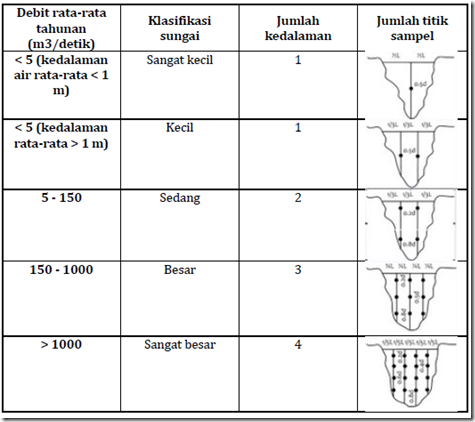" /> Determination River Water Sampling - TNeutron
Home > English > Determination River Water Sampling

# Determination River Water Sampling

If the capture location has been set, the next step is to determine the point of uptake. The number of points is highly dependent on the annual average discharge and classification of the river. A growing number of sampling points, the indescribable quality of the actual river water. Table 12 below illustrates the amount of river water withdrawal points corresponding classification.

Table 13. The number of sampling points of river water according classificationNote: (*) river water samples taken at 30 cm below the water level and / or 30 cm above the bottom of the river and had to be careful so that the riverbed sediment (sediment) is not drawn

Determination of sampling points of water intended that at the time of sampling, objects that float on the surface of the water and sediment that may be eroded from the riverbed is not fetched. Water sampling point in the form of surface water set out under the following conditions:

• On the river with a discharge of less than 5 m3 / sec, water samples are taken at one point in the middle of the river at 0.5 x depth of the river.
• At the river by discharge between 5-150 m3 / sec, water samples were taken from two points, each at a distance of 1/3 and 2/3 the width of the river at 0.5 x depth of the river.
• On the river with a discharge of more than 150 m3 / sec, the water samples taken a minimum of 6 points, each at a distance of ¼, ½, and ¾ the width of the river, at a depth of 0.2 x 0.8 x depth of the river and the river

In practice, the number of points is greatly influenced by the situation and the condition of the river water. For a more detailed overview, Table 13 below shows the number of sampling points of river water discharge based on the classification and the annual average.

Table 14. The number of sampling points of river water discharge based on the classification and the annual averageDescription:
d: depth of the river; L: the width of the river